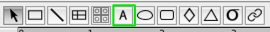Any text box within the FlowJo’s layout editor is capable of evaluating dynamic equations. These may be simple sums or ratios of statistics, or can be arbitrarily complex algebra formulas.

To create a text box, simply click on the text tool in the toolbar.You can also just drag a statistic in to a layout from the workspace. This will create a text box. You can drag multiple statistics into the text box, like in the use case below where both the CD4+ Count and CD8+ Count were added.Here,  I typed in the “Ratio of CD4/CD8:” (without the quotations) and copy/pasted the statistic values from the CD4+ and CD8+ Count (you don’t have to copy/paste and can simply use the values as they come into the text box, but for this demonstration, it is easier to show where those statistics used in the equation came from).

HINT: Hold down the Control key while dragging the statistics into your equation to leave out the label.

Now, select the created equation and right click.Select Equation from Selection and see the results:You can use simple expressions like statistic of your choice * 2, or complex algebraic expressions. You can free type, or use statistics in the equations as in the example above.